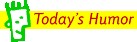Front page > Lesson Plans > Math > General > Tricks for Your Digits We've all heard of the different tricks that can help students learn their multiplication tables. We're doing our best to compile those tricks here. We welcome your contributions! Just send us an email so we can add your trick - be sure to tell us who you are. Trick for multiplying by 6, 7, 8, and 9 (from T2T contributor, John Sipp) With an easy to read (and apply) marker or pen, write the following numbers on both of your hands: put a 9 on each of your index fingers, an 8 on your middle fingers, 7 on your ring fingers, and 6 on your pinkies.Hold your hand out in front of you as if you are going to clap them together, fingers parallel to each other and the floor. Touch the fingers together that are numbered with the digits you're multiplying. Now count the two fingers that are touching, and any fingers that are physically below those two fingers (all the fingers closer to the floor.) Multiply this number by 10. (Example: for 7 x 8, count the two fingers that are touching, and the remaining pinky on one hand, and pinky & ring finger of the other hand for a total of 5, so 50.) Next, count the fingers that are remaining on each hand, above the two fingers touching together. (For 7 x 8, you will count the thumb, index, and middle finger of one hand for 3, and the thumb and index finger of the other hand for 2.) Now multiply these two digits together. (3 x 2 = 6) So take your first number, 50, and add the second number to it, 6, for a total of 56 - the answer to 7 x 8! We realize, this sounds a little complicated, but once you've done it two or three times, it makes perfect sense. It won't be long at all before you don't need the numbers to be written on your hands! Finger Math for 9's (from T2T contributor, Sally Engle) "This may be an easier way to do 9's with finger math. Choose the number you will multiply by nine. Count to that number beginning with the pinky finger of the left hand with palms facing down. Once you get to that number, fold that finger down. The numbers to the left of the folded finger are tens. The numbers to the right of the folded finger are ones. Example: 9 x 7 = 63 Count to seven starting with the left pinkie finger. That should put you to the pointer finger of the right hand. Fold that pointer finger under. To the left you have six digits or 60. To the right you have 3 fingers or 3. 63!" Finger Math for 9's - take 2 (from T2T contributor, Veronica Leigh) "I have struggled with the multiplication tables since grade four. Here is a trick I use for the nines: Subtract 1 from the number that's not 9 and that is the first number of your product. Then, whatever number it takes to add to 9 is your second number. Here's an example for 7 X 9: ( 7 - 1 = 6) and (6 + 3 = 9) Therefore, 7X9 is 63! I am taking my math methods class right now, and my instructor left an interesting note on one of my assignments that was returned today. It said that by grade 5, only 80% of children can think multiplicatively. I don't know the source, but I'll have to find out. I thought it was interesting..." FingerLESS Math for 9's (from T2T contributor, Luann) "The answer for multiplying 9 always adds to nine. (9x9=81) Take the number other than 9, which is 9 in this case go one less for the first number in the answer 8 and ask 8+?=9, 8+1=9- so your answer is 81 9x8=? 8-1=7, and 7+2=9 so 72 is the answer. 9x7=? 7-1=6, 6+3=9 so your answer is 63. It makes sense to me! And was easier for me than the counting with the fingers. "History Lesson From T2T contributor Paul F. Grant, Middle School Teacher, Phoenix, AZ Mahatma Gandhi, as you know, walked barefoot most of the time, which produced an impressive set of callouses on his feet. He also ate very little, which made him rather frail and with his odd diet, he suffered from bad breath. This made him what?     A super calloused fragile mystic plagued with halitosis.